Note

This page is a reference documentation. It only explains the function signature, and not how to use it. Please refer to the user guide for the big picture.

# nilearn.plotting.plot_glass_brain#

nilearn.plotting.plot_glass_brain(stat_map_img, output_file=None, display_mode='ortho', colorbar=False, cbar_tick_format='%.2g', figure=None, axes=None, title=None, threshold='auto', annotate=True, black_bg=False, cmap=None, alpha=0.7, vmin=None, vmax=None, plot_abs=True, symmetric_cbar='auto', resampling_interpolation='continuous', radiological=False, **kwargs)[source]#

Plot 2d projections of an ROI/mask image (by default 3 projections: Frontal, Axial, and Lateral). The brain glass schematics are added on top of the image.

The plotted image should be in MNI space for this function to work properly.

Only glass brain can be plotted by switching stat_map_img to None.

Parameters:
stat_map_imgNiimg-like object

See Input and output: neuroimaging data representation. The statistical map image. It needs to be in MNI space in order to align with the brain schematics.

output_file`str`, or None, optional

The name of an image file to export the plot to. Valid extensions are .png, .pdf, .svg. If output_file is not None, the plot is saved to a file, and the display is closed.

display_modestring, default=’ortho’

Choose the direction of the cuts: ‘x’ - sagittal, ‘y’ - coronal, ‘z’ - axial, ‘l’ - sagittal left hemisphere only, ‘r’ - sagittal right hemisphere only, ‘ortho’ - three cuts are performed in orthogonal directions. Possible values are: ‘ortho’, ‘x’, ‘y’, ‘z’, ‘xz’, ‘yx’, ‘yz’, ‘l’, ‘r’, ‘lr’, ‘lzr’, ‘lyr’, ‘lzry’, ‘lyrz’.

colorbar`bool`, optional

If True, display a colorbar on the right of the plots. Default=False.

cbar_tick_format: str, default=”%.2g” (scientific notation)

Controls how to format the tick labels of the colorbar. Ex: use “%i” to display as integers.

figure`int`, or `matplotlib.figure.Figure`, or None, optional

Matplotlib figure used or its number. If None is given, a new figure is created.

axes`matplotlib.axes.Axes`, or 4 tupleof `float`: (xmin, ymin, width, height), default=None

The axes, or the coordinates, in matplotlib figure space, of the axes used to display the plot. If None, the complete figure is used.

title`str`, or None, default=None

The title displayed on the figure.

thresholda number, None, or ‘auto’, optional

If None is given, the image is not thresholded. If a number is given, it is used to threshold the image: values below the threshold (in absolute value) are plotted as transparent. If “auto” is given, the threshold is determined magically by analysis of the image. Default=’auto’.

annotate`bool`, default=True

If annotate is True, positions and left/right annotation are added to the plot.

black_bg`bool`, or “auto”, optional

If True, the background of the image is set to be black. If you wish to save figures with a black background, you will need to pass facecolor=”k”, edgecolor=”k” to `matplotlib.pyplot.savefig`. Default=False.

cmap`matplotlib.colors.Colormap`, or `str`, optional

The colormap to use. Either a string which is a name of a matplotlib colormap, or a matplotlib colormap object. Default=None.

alphafloat between 0 and 1, default=0.7

Alpha transparency for the brain schematics.

vmin`float`, optional

Lower bound of the colormap. If None, the min of the image is used. Passed to `matplotlib.pyplot.imshow`.

vmax`float`, optional

Upper bound of the colormap. If None, the max of the image is used. Passed to `matplotlib.pyplot.imshow`.

plot_absboolean, default=True

If set to True maximum intensity projection of the absolute value will be used (rendering positive and negative values in the same manner). If set to false the sign of the maximum intensity will be represented with different colors. See Glass brain plotting in nilearn (all options) # noqa for examples.

symmetric_cbar`bool`, or “auto”, default=”auto”

Specifies whether the colorbar and colormap should range from -vmax to vmax (or from vmin to -vmin if -vmin is greater than vmax) or from vmin to vmax. Setting to “auto” (the default) will select the former if either vmin or vmax is None and the image has both positive and negative values.

resampling_interpolation`str`, optional

Interpolation to use when resampling the image to the destination space. Can be:

• “continuous”: use 3rd-order spline interpolation

• “nearest”: use nearest-neighbor mapping.

Note

“nearest” is faster but can be noisier in some cases.

Default=’continuous’.

radiological`bool`, default=False

Invert x axis and R L labels to plot sections as a radiological view. If False (default), the left hemisphere is on the left of a coronal image. If True, left hemisphere is on the right.

Returns:
display`OrthoProjector` or None

An instance of the OrthoProjector class. If `output_file` is defined, None is returned.

Notes

Arrays should be passed in numpy convention: (x, y, z) ordered.

## Examples using `nilearn.plotting.plot_glass_brain`#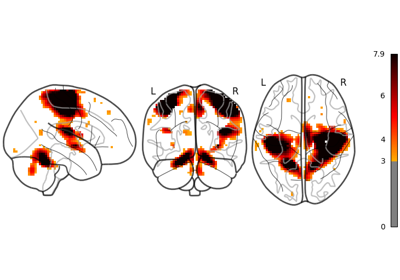Glass brain plotting in nilearn

Glass brain plotting in nilearn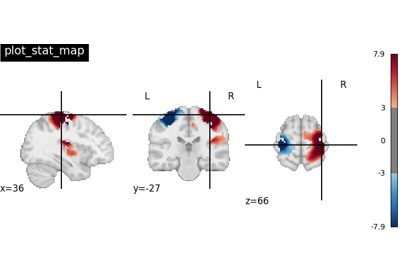Plotting tools in nilearn

Plotting tools in nilearn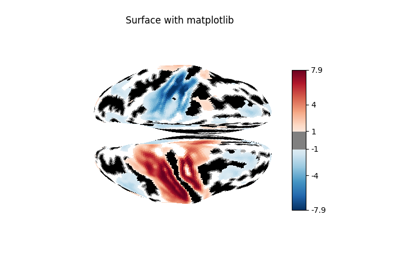Making a surface plot of a 3D statistical map

Making a surface plot of a 3D statistical mapGlass brain plotting in nilearn (all options)

Glass brain plotting in nilearn (all options)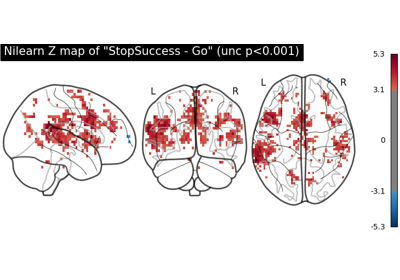First level analysis of a complete BIDS dataset from openneuro

First level analysis of a complete BIDS dataset from openneuro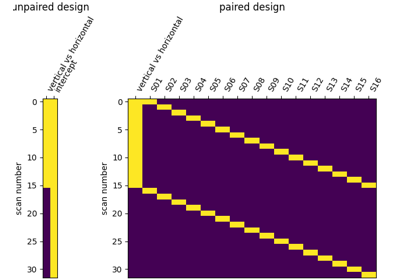Second-level fMRI model: two-sample test, unpaired and paired

Second-level fMRI model: two-sample test, unpaired and paired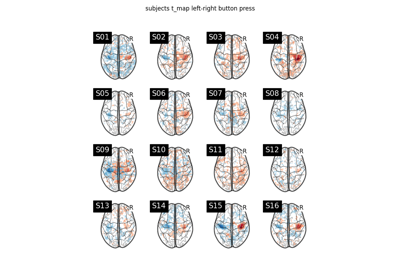Second-level fMRI model: one sample test

Second-level fMRI model: one sample test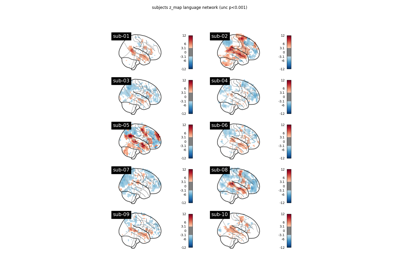BIDS dataset first and second level analysis

BIDS dataset first and second level analysis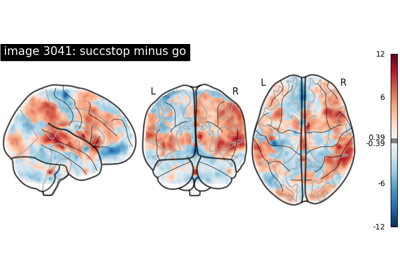NeuroVault meta-analysis of stop-go paradigm studies

NeuroVault meta-analysis of stop-go paradigm studies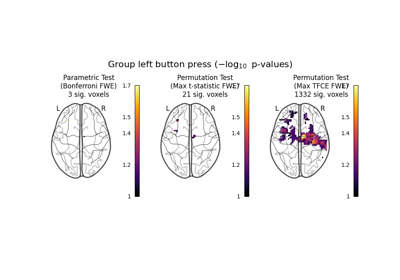Massively univariate analysis of a motor task from the Localizer dataset

Massively univariate analysis of a motor task from the Localizer dataset